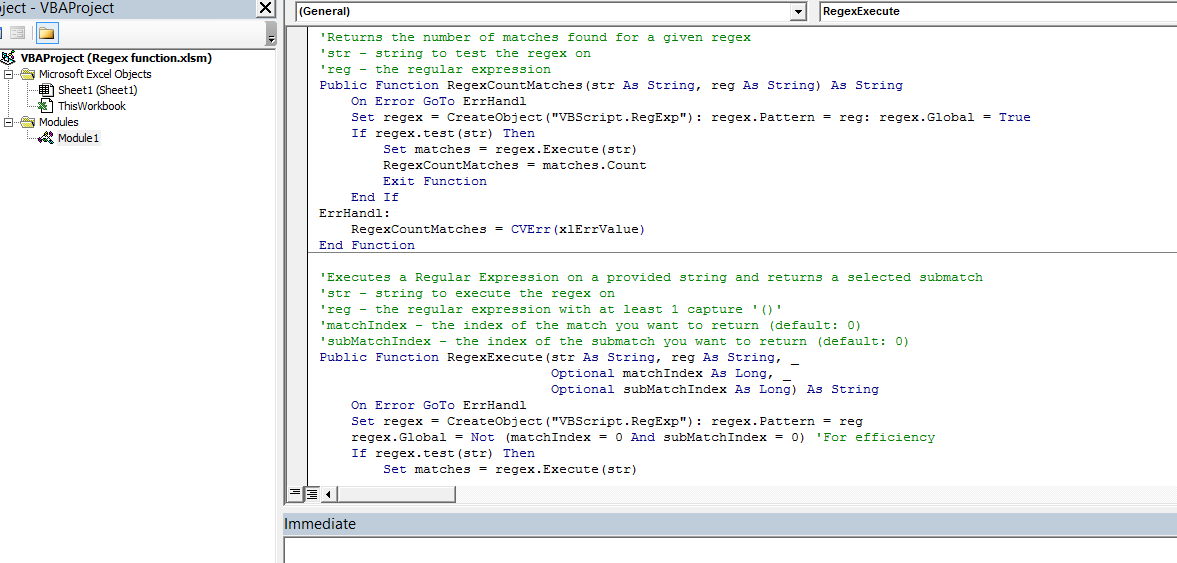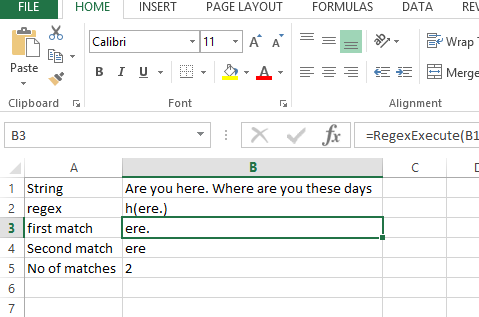## How it works?

Excel does not natively provide any regex functions, which often requires creating complex formulas for extracting pieces of strings otherwise easy to extract using Regular Expressions. Hence, to facilitate Regex in Excel you need to use User Defined Functions (UDFs) – functions defined in VBA but accessible as regular functions in Excel.

Below you will find 2 basic UDF functions created just for this use:

Let us write the following function in Excel and save the file as a macro-enabled file:## The VBA code

You can copy from here as well, or you can check the file attached at the end:

```'Returns the number of matches found for a given regex
'str - string to test the regex on
'reg - the regular expression
Public Function RegexCountMatches(str As String, reg As String) As String
On Error GoTo ErrHandl
Set regex = CreateObject("VBScript.RegExp"): regex.Pattern = reg: regex.Global = True
If regex.test(str) Then
Set matches = regex.Execute(str)
RegexCountMatches = matches.Count
Exit Function
End If
ErrHandl:
RegexCountMatches = CVErr(xlErrValue)
End Function```

‘Executes a Regular Expression on a provided string and returns a selected submatch
‘str – string to execute the regex on
‘reg – the regular expression with at least 1 capture ‘()’
‘matchIndex – the index of the match you want to return (default: 0)
‘subMatchIndex – the index of the submatch you want to return (default: 0)
Public Function RegexExecute(str As String, reg As String, _
Optional matchIndex As Long, _
Optional subMatchIndex As Long) As String
On Error GoTo ErrHandl
Set regex = CreateObject(“VBScript.RegExp”):
regex.Pattern = reg regex.Global = Not (matchIndex = 0 And subMatchIndex = 0) ‘For efficiency
If regex.test(str) Then
Set matches = regex.Execute(str)
RegexExecute = matches(matchIndex).SubMatches(subMatchIndex)
Exit Function
End If ErrHandl:
RegexExecute = CVErr(xlErrValue)
End Function

Now you can check this in the Excel file like this:

Using formulas :

first match: =RegexExecute(B1,B2,0)

Second match: =RegexExecute(B1,B2,1)

No of matches: =RegexCountMatches(B1,B2)## Repeating Patterns

Regex allows you to work with repeating patterns of text. This is useful when you have structured data, such as a list of email addresses, and you want to extract specific elements.

Let’s say you have a list of email addresses in cell A1, separated by commas. You want to extract all the domains (e.g., “@example.com”) from the list. You can use the following formula:

=RegexExecute(A1, “(\S+@\S+)”, 0, 1)

(\S+@\S+): This pattern captures email addresses.

The formula extracts all email addresses from the text, even if they are separated by commas. It’s a practical way to work with repeating patterns in structured data.

Regex empowers you to manipulate text data effectively. These techniques expand your capabilities in data processing and analysis, making Excel a versatile tool for text manipulation.